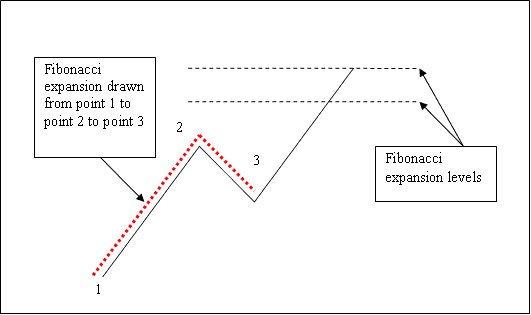# Indices Trading Fibonacci Extension Indicator Example

To Calculate Indices Trading Fibonacci Extension levels on indices charts we use the indices trading Indices Trading Fibonacci Extension Indicator

What are the Indices Trading Fibonacci Extension Levels?

• 61.8% Indices Trading Fibonacci Extension Level

• 100% Indices Trading Fibonacci Extension Level

We use Fibonacci expansionlevels to estimate where the stock indexes price movement will reach. There are 2 important fibonacci expansion levels; 61.8% and 100% Indices Trading Fibonacci Extension levels, these level are used for taking profit.

To draw Fibonacci expansion we use 3 chart points.

To draw Fibonacci extension levels we wait until the stock indexes price retracement is complete and indices price starts to move in the original direction of the Indices trend. Where the retracement reaches is used as point 3.

The example explained and illustrated below shows the 3 Chart Points where the Fibonacci extension indicator is drawn, marked as chart point 1, 2 and 3. Chart point 1 is where the stock indexes price indices trend started, Chart point 2 is where the stock indexes price indices trend retraced and chart point 3 is where the stock indexes price retracement reached as shown on the stock indexes trading example explained and illustrated below.Indices Trading Fibonacci Extension Indicator Explained

The two Fibonacci extension levels 61.8% and 100% Indices Trading Fibonacci Extension levels were then drawn above the Indices Trading Fibonacci Extension Indicator as shown on the stock indexes trading example above.